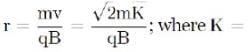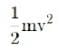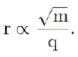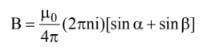# Assertion & Reason Test: Moving Charges & Magnetism - 1

## 10 Questions MCQ Test Topic-wise MCQ Tests for NEET | Assertion & Reason Test: Moving Charges & Magnetism - 1

Description
Attempt Assertion & Reason Test: Moving Charges & Magnetism - 1 | 10 questions in 20 minutes | Mock test for NEET preparation | Free important questions MCQ to study Topic-wise MCQ Tests for NEET for NEET Exam | Download free PDF with solutions
QUESTION: 1

### Directions: In the following questions, A statement of Assertion (A) is followed by a statement of Reason (R). Mark the correct choice as.Assertion (A): Magnetic field interacts with a moving charge only.Reason (R): Moving charge produces a magnetic field.

Solution: Current carrying wire creates a magnetic field. This magnetic field has no effect on a stationary charge. But when the charge also moves, it creates a current. This current produces a magnetic field. Two fields interact and the charge is deflected. So, the assertion is true. Moving charge creates a current which produces a magnetic field. So, the reason is also true. Reason is the correct explanation of A.
QUESTION: 2

### Directions: In the following questions, A statement of Assertion (A) is followed by a statement of Reason (R). Mark the correct choice as.Assertion (A): The energy of a charged particle moving in a uniform magnetic field remains constant.Reasoning (R): Work done by the magnetic field on the charge is zero.

Solution: The force on a charged particle moving in a uniform magnetic field always acts in direction perpendicular to the direction of motion of the charge. So work done by the magnetic field, W = FS cos θ = FS cos 90° = 0 So, the energy of the charged particle does not change. Both assertion and reason are true and reason also explains the assertion.
QUESTION: 3

### Directions: In the following questions, A statement of Assertion (A) is followed by a statement of Reason (R). Mark the correct choice as.Assertion (A): The magnetic field at the ends of a very long current carrying solenoid is half of that at the centre.Reason (R): Magnetic field within a sufficiently long solenoid is uniform.

Solution: Magnetic field inside a solenoid is B = μ0ni. Magnetic field at the end of a solenoid is 1/2 μ0ni. So, the assertion is true. Magnetic field within a sufficiently long solenoid is uniform. So the reason is also true. But it does not explain the assertion.
QUESTION: 4

Directions: In the following questions, A statement of Assertion (A) is followed by a statement of Reason (R). Mark the correct choice as.

Assertion (A): A direct current flowing through a metallic rod produces magnetic field both inside and outside of the rod.

Reason (R): There is no flow of charge carrier inside the rod.

Solution: Charge carries flows through the whole cross-section. So, the filed exists both inside and outside. So, the assertion is true and the reason is false.
QUESTION: 5

Directions: In the following questions, A statement of Assertion (A) is followed by a statement of Reason (R). Mark the correct choice as.

Assertion (A): Torque on a coil is maximum when it is suspended radially in a magnetic field.

Reason (R): Torque tends to rotate a coil.

Solution: The torque on the coil in a magnetic field is given by τ = nIBA sin θ For radial field, θ = 90° and sin θ = 1 Torque = nIBA and it is maximum. So the assertion is true. Torque is the rotational equivalence of force.

So, torque will tend to rotate a coil. Reason is also true. But reason cannot explain the assertion that why the torque is maximum in the specified position.

QUESTION: 6

Directions: In the following questions, A statement of Assertion (A) is followed by a statement of Reason (R). Mark the correct choice as.

Assertion (A): Earth’s magnetic field does not affect the functioning of a moving coil galvanometer.

Reason (R): Earth’s magnetic field is too weak.

Solution: The coil of the moving coil galvanometer is suspended in a very strong radial magnetic field. Earth’s magnetic field is too weak compared to that and hence its effect is negligible. So, assertion and reason both are true and the reason explains the assertion properly.
QUESTION: 7

Directions: These questions consist of two statements, each printed as Assertion and Reason. While answering these questions, you are required to choose any one of the following four responses.

Assertion : Cyclotron does not accelerate electrons.

Reason : Mass of the electrons is very small.

Solution: A cyclotron is a kind of particle accelerator invented by Ernest O. Lawrence at the University of California, Berkeley, in 1929–1930, and patented in 1932. A cyclotron accelerates charged particles along a spiral path outward from the center. The particles are guided by a static magnetic field to a spiral trajectory, and accelerated by a rapidly changing electric field (radio frequency). For this invention Lawrence received the 1939 Nobel Prize in Physics.

Cyclotrons were the most efficient particle accelerator equipment until the 1950s, when the synchrotron replaced them, and are now used in science and nuclear medicine to generate particle beams.

This will toss the particle out of resonance with the oscillating field which means that when the particle reaches the hole between the dees, the extremity of the dees won't change. Thus, the particle won't be accelerated further.

So, Cyclotron isn't reasonable for accelerating electrons. Because of the small mass, the speed of electrons increments quickly. Similarly, because of fast relativistic variation in their mass, the electrons escape step with the oscillating electric field.

Hence, option A is the correct option.

QUESTION: 8

Directions: These questions consist of two statements, each printed as Assertion and Reason. While answering these questions, you are required to choose any one of the following four responses.

Assertion: The magnetic field produced by a current carrying solenoid is independent of its length and cross-sectional area.

Reason: The magnetic field inside the solenoid is uniform.

Solution: Magnetic field inside the solenoid at point P is given by, B=μ0/4π (2πni)[sinα+sinβ] Where n=no. of turns per unit length =N/l. Thus it is clear that magnetic field is independent of length and cross sectional area. Also the magnetic field with in the solenoid is uniform and parallel to the axis of the solenoid.
QUESTION: 9

Directions: These questions consist of two statements, each printed as Assertion and Reason. While answering these questions, you are required to choose any one of the following four responses.

Assertion : A proton and an alpha particle having the same kinetic energy are moving in circular paths in a uniform magnetic field. The radii of their circular paths will be equal.

Reason : Any two charged particles having equal kinetic energies and entering a region of uniform magnetic field B in a direction perpendicular to B, will describe circular trajectories of equal radii.

Solution: The radius of the circular path is given bySince K and B are the same for the two particles,Now, the charge of an alpha particle is twice that of a proton and its mass is four times the mass of a proton, √ m /q will be same for both particles.

Hence, r will be the same for both particles.

QUESTION: 10

Directions: These questions consist of two statements, each printed as Assertion and Reason. While answering these questions, you are required to choose any one of the following four responses.

Assertion : The magnetic field at the centre of the circular coil in the following figure due to the currents I1 and I2 is zero.

Reason : I1 = I2 implies that the fields due to the current I1 and I2 will be balanced.

Solution:

The magnetic field inside the solenoid at point
P is given by,where n = no. of turns per unit length = N/l
Thus it is clear that mangetic field is
independent of length and cross sectional
Also the magnetic field with in the solenoid
is uniform and parallel to the axis of the
solenoid.Use Code STAYHOME200 and get INR 200 additional OFF Use Coupon Code

### How to Prepare for NEET

Read our guide to prepare for NEET which is created by Toppers & the best Teachers